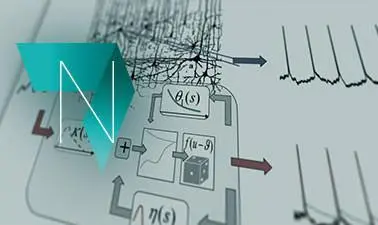There is 0 item now in your comparison listView a comparison list

# Neuronal Dynamics

Price
\$139
19,545 people completed this program

## Overview

This course gives an introduction to the field of theoretical and computational neuroscience with a focus on models of single neurons. Neurons encode information about stimuli in a sequence of short electrical pulses (spikes). Students will learn how mathematical tools such as differential equations, phase plane analysis, separation of time scales, and stochastic processes can be used to understand the dynamics of neurons and the neural code.

Week 1: A first simple neuron model

Week 2: Hodgkin-Huxley models and biophysical modeling

Week 3: Two-dimensional models and phase plane analysis

Week 4: Two-dimensional models (cont.)/ Dendrites

Week 5: Variability of spike trains and the neural code

Week 6: Noise models, noisy neurons and coding

Week 7: Estimating neuron models for coding and decoding

Before your course starts, try the new edX Demo where you can explore the fun, interactive learning environment and virtual labs. Learn more.

• How mathematical tools such as differential equations, phase plane analysis, separation of time scales, and stochastic processes can be used to understand the dynamics of neurons and the neural code

## Authored by

École polytechnique fédérale de Lausanne

## Reviews(0)

Help others make their choice. Be the first one to leave a review

Leave a reviewBeginner
English
7 weeks
Self-paced
Online